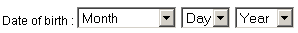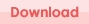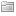## Date control using JavaScript

Date control is a combination of three drop downs with month, day and year valus. It can be used as an alternative to the date picker control. Suppose a user needs to select a date before/after 10 years. At this point selecting the date with a date picker will not be easy and time consuming. So, in this situation, this control may be helpful.To customize you need you can configure it. Change the properties in date_control.js file.

How to use it?

2. Link the date_control to the page you want to use it in.
3. Write this code where you want to place the control

```<script language="javascript"> // getting the date control getDateControl("name_of_the_field", "name_of_the_form"); </script>```

I have tested the code with Internet Explorer 6 and FireFox 2.X.X and it works fine.

You can download a copy from here:JavaScript, My Works

## Paging using PHP and MySQL

I have coded a class for paging, you may find it here.

```### Code for Paging Starts ### //index no of the page \$pageIndex = ((int)\$_REQUEST['pageIndex'] == 0) ? 1 : (int)\$_REQUEST['pageIndex'];   //number of rows \$rowPerPage = 10;   //counting total number of rows \$sql = 'SELECT COUNT(*)         FROM {your_table_name}         WHERE {IF ANY CONDITION}';   \$query = mysql_query(\$sql, \$link_identifier); \$row = mysql_fetch_row(\$query);   // total records \$totalRecords = \$row;   // number of pages \$totalPages = ceil(\$totalRecords/\$rowPerPage);   if(\$pageIndex > \$totalPages) {     \$pageIndex = \$totalPages; }   \$startIndex = (\$pageIndex - 1) * \$rowPerPage;   if(\$totalRecords > \$rowPerPage) {     \$endIndex = \$startIndex + \$rowPerPage; } else {     \$endIndex = \$totalRecords; }   if(\$pageIndex == \$totalPages) {     if(\$totalRecords > \$totalPages * \$rowPerPage)     {         \$endIndex = \$totalRecords;     } }   if(\$totalRecords > 0) {     \$rangeStart = \$startIndex+1;       if((\$totalRecords > \$rowPerPage) || (\$pageIndex == \$totalPages))     {         \$rangeEnd = \$totalRecords;     }     else     {         \$rangeEnd = \$endIndex;     }       \$range = 'Showing '  . \$rangeStart.' - '.\$rangeEnd.' of '.\$totalRecords; }   // link for next page \$nextLink = 'page_name.php?pageIndex='.(\$pageIndex + 1);   // link for previous page \$previousLink = 'page_name.php?pageIndex='.(\$pageIndex - 1);   // fetching data using pagination \$sql = "SELECT *             FROM {your_table_name}             WHERE {IF ANY CONDITION}             LIMIT \$startIndex, \$rowPerPage";   \$query = mysql_query(\$sql, \$link_identifier);   ### Code for Paging Ends ###```

Now use the resource ‘\$query’ and do what you want to do.PHP

blog